4901.0 - Children's Participation in Cultural and Leisure Activities, Australia, Apr 2009
Previous ISSUE Released at 11:30 AM (CANBERRA TIME) 28/10/2009
Page tools:Print AllRSSSearch this Product

TECHNICAL NOTE DATA QUALITY

RELIABILITY OF ESTIMATES

1 Since the estimates in this publication are based on information obtained from occupants of a sample of dwellings, they are subject to sampling variability; that is, they may differ from those that would have been produced if all dwellings had been included in the survey. One measure of the likely difference is given by the standard error (SE), which indicates the extent to which an estimate might have varied by chance because only a sample of dwellings was included. There are about two chances in three (67%) that a sample estimate will vary by less than one SE from the number that would have been obtained if all dwellings had been included, and about 19 chances in 20 (95%) that the difference will be less than two SEs.

2 Another measure of the likely difference is the relative standard error (RSE), which is obtained by expressing the SE as a percentage of the estimate.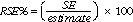3 Space does not allow for the separate indication of the SEs and/or RSEs of all the estimates in this publication. However, RSEs were calculated for each separate estimate and are available to download free-of-charge as Excel spreadsheets from the ABS website <www.abs.gov.au> as an attachment to this publication. The Jackknife method of variance estimation is used to calculate SEs, which involves the calculation of 30 replicate estimates based on 30 different sub samples of the original sample. The variability of estimates obtained from these sub samples is used to estimate the sample variability surrounding the main estimate.

4 In the tables in this publication, only estimates (numbers or percentages) with RSEs less than 25% are considered sufficiently reliable for most purposes. However, estimates with larger RSEs have been included and are preceded by an asterisk (e.g.*3.4) to indicate they are subject to high SEs relative to their estimate and should be used with caution. Estimates with RSEs greater than 50% are preceded by a double asterisk (e.g.**2.1) to indicate that they are considered too unreliable for general use.

CALCULATING STANDARD ERRORS FOR POPULATION ESTIMATES

5 Standard errors can be calculated using the estimates (counts or proportions) and their corresponding RSEs. For example, Table 5 shows the estimated number of children who participated in playing a musical instrument was 535,400. The RSE Table corresponding to the estimates in Table 5 (see Table 5 Relative Standard Errors in the 'Relative Standard Error' section at the end of these Technical Notes) shows the RSE for this estimate is 3.1%. The SE is calculated by: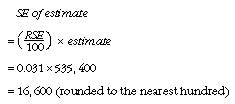6 Therefore, there are about two chances in three that the value that would have been produced if all children had been included in the survey will fall within the range 518,800 to 552,000 and about 19 chances in 20 that the value will fall within the range 502,200 to 568,600. This example is illustrated in the diagram below: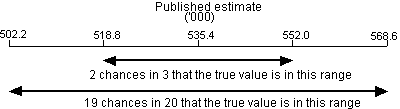7 In general, the size of the SE increases as the size of the estimate increases. Conversely, the RSE decreases as the size of the estimate increases. Very small estimates are subject to such high RSEs that their value for most practical purposes is unreliable and should only be used to aggregate with other estimates to provide derived estimates with RSEs of less than 25%.

CALCULATING STANDARD ERRORS FOR MEANS AND MEDIANS

8 This publication contains means and medians. Both are measures for locating the centre of a set of values, but each measure has its own method of calculation. The mean is the arithmetic average, whereas the median is the middle value of a set of values when the values are sorted in size order.

9 Table 5 shows that the estimated number of children who played a musical instrument was 535,400, with the median number of times those children played a musical instrument being 48 times in the last 12 months.

10 Standard errors can be calculated using the estimates (means or medians) and their corresponding RSEs. The RSE Table corresponding to the estimates in Table 5 (see Table 5 Relative Standard Errors in the 'Relative Standard Error' section at the end of these Technical Notes) shows the RSE for the estimated median number of times children played a musical instrument in the last 12 months is 5.3%. The SE is calculated by: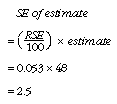11 Therefore, there are about two chances in three that the value that would have been produced if all children had been included in the survey will fall within the range 45.5 and 50.5 and about 19 chances in 20 that the value will fall within the range 43 to 53. This example is illustrated in the diagram below: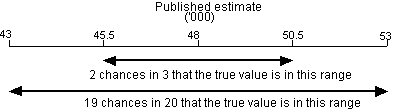CALCULATING STANDARD ERRORS FOR DIFFERENCES OF ESTIMATES

12 The difference between two survey estimates (numbers or percentages) is also subject to sampling error. The sampling error of the difference between two estimates depends on their SEs and the relationship (correlation) between them. An approximate SE of the difference between two estimates (x-y) may be calculated by the formula: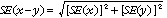13 While this formula will only be exact for differences between separate and uncorrelated (unrelated) characteristics of sub-populations, it is expected to provide a good approximation for all differences likely to be of interest in this publication.

SIGNIFICANCE TESTING

14 The statistical significance test for any of the comparisons between estimates was performed to determine whether, with a certain level of confidence, there is a true difference between the corresponding population characteristics. The standard error of the difference between two corresponding estimates (x and y) can be calculated using the formula in paragraph 12. This standard error is then used to calculate the following test statistic: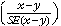15 If the value of this test statistic is greater than 2 then there is good evidence of a real difference in the two populations with respect to that characteristic. Otherwise, it cannot be stated with confidence that there is a real difference between the populations.

RELATIVE STANDARD ERROR

16 Relative Standard Errors for Table 5 are included below. However, RSEs for all tables are available free-of-charge on the ABS website <www.abs.gov.au>, released in spreadsheet format as an attachment to this publication.

 TABLE 5 RSEs: CHILDREN PARTICIPATING IN ORGANISED CULTURAL ACTIVITIES(a), Frequency in last 12 monthsPlaying a musical instrument Singing Dancing Drama RSE of number (%) 1-13 times 8.7 8.6 6.8 12.0 14-26 times 8.2 15.5 10.6 19.3 27-52 times 5.1 12.8 6.7 15.5 53-104 times 11.2 27.0 10.2 47.6 105 times or more 6.9 35.4 12.0 72.7 Total participants 3.1 6.0 3.4 8.4 RSE of proportion (%) 1-13 times 8.1 5.4 5.9 10.5 14-26 times 8.1 15.7 10.2 16.0 27-52 times 4.7 10.9 5.8 12.4 53-104 times 10.1 27.4 9.4 46.8 105 times or more 5.7 35.1 11.4 71.9 Total participants - - - - RSE of mean and median times (%) Mean 4.7 7.6 5.7 9.9 Median 5.3 12.6 6.3 19.3 - nil or rounded to zero (including null cells) (a) Children aged 5 to 14 years who participated in selected organised cultural activities outside of school hours during the 12 months prior to interview in April 2009.